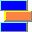# Erlang C Queueing Model (M/M/n)

For general concepts of queueing theory that apply to this queueing model, see About Queueing Models.

## What is the Erlang C Queueing Model?

In the Erlang C queueing model, customers arrive at a queueing system having n servers and infinitely many waiting positions.  We also assume Poisson arrivals and exponentially distributed service times.  Therefore, in Kendall notation, this model is denoted M/M/n/∞, or simply M/M/n

Since there are infinitely many waiting positions, the waiting positions can never be exhausted.  Therefore, every arriving customer will eventually be served, even if after a long wait.  Note that in contrast to the Erlang B queueing model, no customer ever experiences blockage!  However, if the traffic load x in erlangs is greater than or equal to the number n of servers, then the system becomes unstable, in the sense that the number of waiting customers grows without limit.

Part of the definition of the Erlang C queue specifies how waiting customers are selected for service: we assume that whenever there exist waiting customers, the longest-waiting customer will always be the next customer to begin service.  In other words, we assume a FIFO queueing discipline.

## The Erlang C Function

The Erlang C Function C(n,x) is defined by

C(n,x) = n*B(n,x) / ( nx*(1−B(n,x)))

where B(n, x) = (xn/n!) / (1 + x + x2/2! + x3/3! + ... + xn/n!) is the Erlang B function. (See Erlang B Queueing Model.)

The Erlang C function computes the probability that an arriving customer in the Erlang C queueing model will find that all servers are busy.  This is the same as the fraction of arriving customers that are delayed (i.e., must wait) before beginning service.  This function is used in computing many other functions in the Erlang Library for Excel.  The Erlang C function occurs in the Erlang Library for Excel under the name ErlcFractionDelayed.

## Definitions and Formulas

### Erlang C Formulas

n = number of servers

λ = Arrival rate

μ = Service rate

x = Traffic intensity measured in erlangs

C = Probability of delay; see ErlcFractionDelayed

AHT = Average Handle Time (average duration of service)

AWA = Average Wait for All customers (customers with zero wait time are included in the average); see ErlcWait, ErlcWait4

AWD = Average Wait for Delayed customers (customers with zero wait time are not included in the average)

T = Average time in system

NW = Average Number of Waiting customers (average number in queue); see ErlcNwaiting, ErlcNwaiting4

NB = Average Number of Busy servers

NS = Average Number of customers in System

UTIL = Utilization fraction (occupancy, average fraction of the time that each server is busy)

P(t) = Probability that the wait time of a customer will be less than or equal to t, where t any non-negative number.  This is the same as the fraction of callers whose wait time is less than or equal to t.  See ErlcFractionOk.

The Erlang C function C(n,x) is defined as

C(n,x) = n*B(n,x) / ( nx*(1−B(n,x)))

where B(n,x) is the Erlang B function. Then

λ/μ < n and

x = λ/μ

C = C(n,x)

AHT = 1/μ

AWA = C / (μ*(nx))
= C*AHT / (nx)

AWD = AHT / (nx)

T = AWA + AHT

NW = C * / (nx)

NB = x

NS = NB + NW
= x + (C*/ (nx))

UTIL = x/n

P(t) = 1 − C*e−(nx)*t/AHT

In these formulas, the quantities C, AHT, etc., are theoretical averages that are approached as a limit under the assumption that the queue operates for a very long period of time without any change to the queue parameters (number of servers, arrival rate, service rate).

## Telecom Application

Call center analysts often use the Erlang C queueing model to help understand the functioning of a group of agents taking incoming calls in a call center.  As in any application of queueing theory, there are three parts that fit together: (1) a queueing model, (2) a real-world system, and a (3) mapping of the queueing model to the real-world system (see About Queueing Models).  In this example, the queueing model is Erlang C, and the real-world system is a group of agents handling calls in a call center.  The mapping of the queueing model to the real-world system looks like this:

 Erlang C Entity Call Center Entity Server Agent Position in the system Trunk Customer Caller Arrival rate Calls arriving per second Average Handle Time Average Talk Time + Average Wrap Time NW Average Queue Length etc.

Clearly some of the assumptions of the Erlang C model are not true for a call center.  For example, there are only a finite number of trunks, hence only a finite number of places where calls can be parked while waiting for service.  But, if the number of trunks is quite large, then as a practical matter, there may be no situation when all trunks are busy, hence the assumption of infinitely many waiting positions might "almost" be true.  Similarly, the assumptions of Poisson arrivals and exponential service times will not hold exactly.  Nevertheless, experience over many decades has shown that using the Erlang C model can give helpful insights into the operation of agent groups in call centers, provided that

(1) there are a large number of trunks (hence many waiting slots),

(2) the assumptions of Poisson arrivals and exponential service times are approximately correct over the period being studied,

(3) wating calls are handled first-come-first-served.

There are many other applications of the Erlang C model—in telecom and in other fields as well.

## See Also

Abstract Micro Systems, Nashville, Tennessee
Contact Abstract Micro Systems